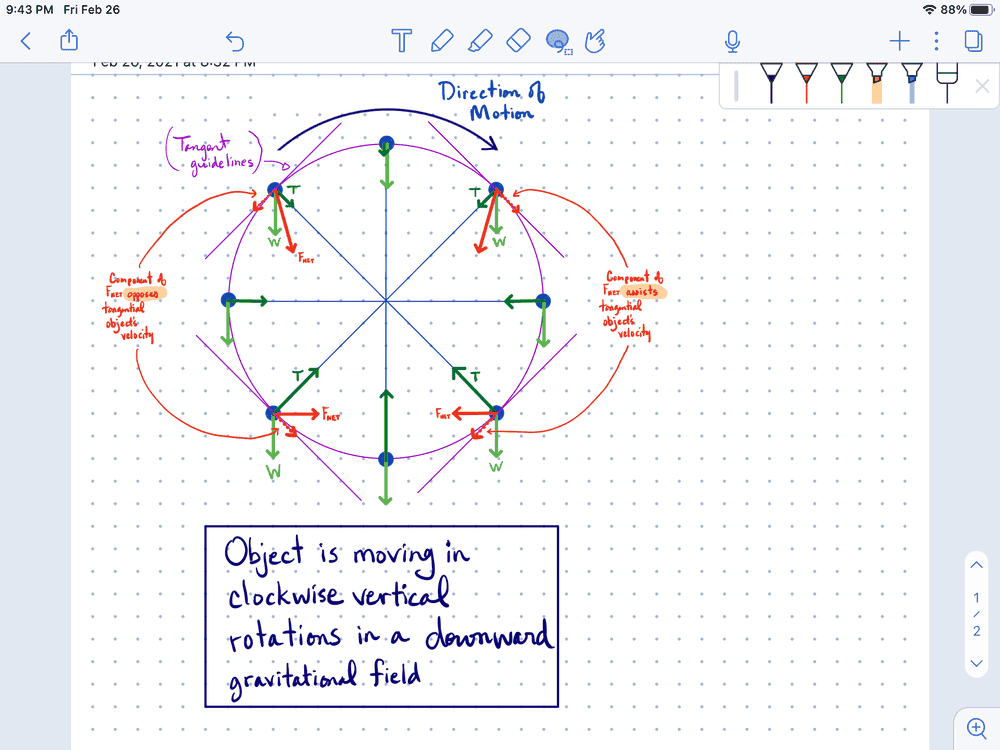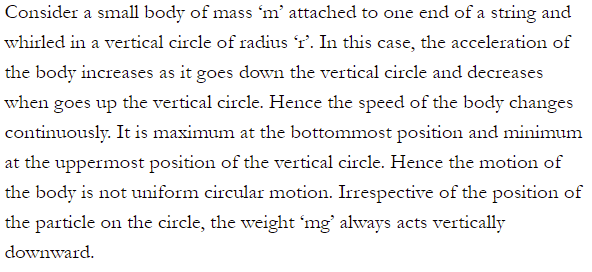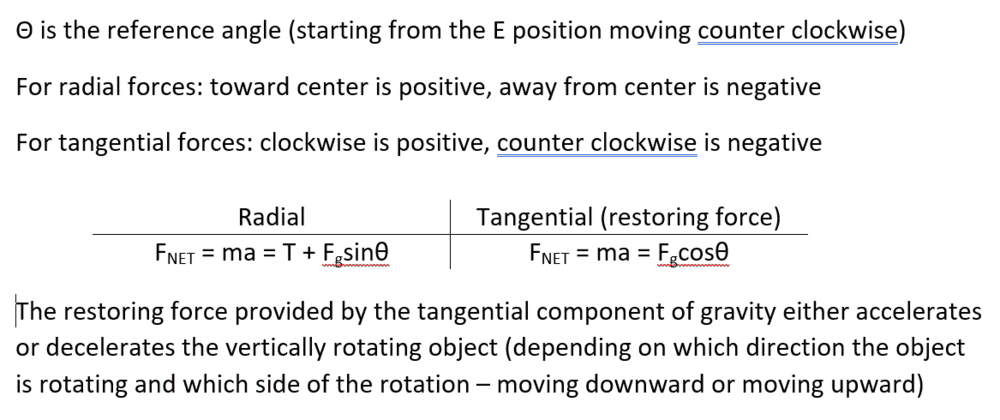# Constant Circular Motion Not Really Constant

John Mohr
I was pondering my practice of talking about circular motion in the horizontal direction and the vertical direction. But I'd often would see in books, notes, and the internet that we assume constant velocity for the vertical case. However, when one thinks about the forces at different points in the circular path we can see from the free body diagrams a net force that may either 'assist' or 'resist' the motion of the object circulating. This would mean that the object would speed up slightly as it descends with the assistance of gravity and slow down slightly with the ascending against gravity. I suppose my thought is that the books, notes, and internet might mean the average speed remains constant as it continues to circulate.

Any thoughts and/or confirmation?

Mentor
How would you analyze it for the more accurate case you have identified?

andresB
The terminology used is weird to me. I would not use the word constant but uniform. And the velocity is not constant, the speed is.

Now, it is true that in a vertical circular motion, like the one of a mass attached to a string, if the only forces are the weight and the tension then the speed will not be constant.

That does not mean you can't have a uniform vertical circular motion, it would just require some compensation forces in order to to have a constant speed/centripetal force.

•PeroK
Homework Helper
Gold Member
To add to what @andresB said, for a mass going around a vertical circle in uniform circular motion, the tension in the string must vary both in magnitude and in direction. Think about it this way.
1. A mass going around a circle at constant speed has constant angular momentum.
2. If the circle is vertical, there is the varying external torque of gravity acting on it.
3. Therefore to keep the angular momentum and hence the speed constant, another external torque must compensate for the gravity torque. This torque can only come from the string.
4. However, a string attached to a fixed point can exert only a radial force which means zero torque. So ##\dots## how does one get out of this?

Answer: Swing a mass at the end of string in a vertical circle and see what your hand holding the string does.

Gold Member
But I'd often would see in books, notes, and the internet that we assume constant velocity for the vertical case.

That is not consistent with my experience. In most of the treatments and examples I see, the motion in vertical circles is not uniform circular motion.

•nasu
John Mohr
How would you analyze it for the more accurate case you have identified?
Thank you to everyone for your comments. I’m sorry I didn’t include a diagram earlier. I got home and finally had a chance to draw a diagram of what I mean.#### Attachments

• Note Feb 26, 2021(1).pdf
731.5 KB · Views: 71
Last edited:
But I'd often would see in books, notes, and the internet that we assume constant velocity for the vertical case.
Can you give an example? It depends on the scenario. For a spinning wheel the speed on the circumference can be constant in the vertical case.

•PeroK
Mentor
Thank you to everyone for your comments. I’m sorry I didn’t include a diagram earlier. I got home and finally had a chance to draw a diagram of what I mean. View attachment 278771
Let's see your Newton's 2nd Law equations for this.

Homework Helper
Gold Member
Thank you to everyone for your comments. I’m sorry I didn’t include a diagram earlier. I got home and finally had a chance to draw a diagram of what I mean. View attachment 278771
Your diagram is faulty. You show two external forces, tension T (blue) and weight W (green). OK. Then you show net force FNet in red. Not OK. The net force must be the vector sum of the blue and green arrows, reasonably drawn to scale. It is not. Examples
At the due East position there should be a read arrow pointing down and to the left. There is none. Why not? Similarly at the due West position.
At the due North position again you show no red arrow. Why not? Both the tension and the weight point down, therefore their red arrow sum must also point down. Similarly at the due South position except that the blue and green arrows point in opposite directions. However, they cannot cancel each other out because there must be net centripetal force to make the mass follow the circular path.

Having said all that, I am not sure under what conditions you drew your diagram. One possibility is that the mass is given a good kick at the due South position and more than enough speed to go around the loop. The other possibility is that the string is replaced by a light rod with some kind of driving mechanism at the center that causes the mass to go around the circle at constant speed. That's the Ferris wheel analogue. The third possibility of a mass at the end of a string going around the circle of fixed center at constant speed is not a possibility for reasons that I explained in post #4.

Gold Member
2022 Award
I think with "vertical circular motion" you mean a pendulum. Its equation of motion is
$$m a \ddot{\theta}=-m g \sin \theta \; \Rightarrow \; \ddot{\theta}=-\frac{g}{a} \sin \theta.$$
The solution is an elliptic function and the angular velocity, ##\dot{\theta}## is for sure not constant.

You can see this of course already in the small-angle approximation, i.e., for ##|\theta|\ll 1##, where you can approximate ##\sin \theta \simeq \theta##, from which you get
$$\ddot{\theta}=-\omega^2 \theta, \quad \omega=\frac{g}{a}.$$
The solution is
$$\theta(t)=A \cos(\omega t + \varphi),$$
and the angular velocity is
$$\dot{\theta}(t)=-A \omega \sin(\omega t+\varphi).$$

John Mohr
Ok, so I will do my best to address each person's comment the best I can.

But, perhaps to start, I thought a visual example might be helpful to explain what I've seen before. I know there is a whole lot more going on with the physics on this example but I'm hoping the general idea will be seen. When we see gymnasts doing 'giants' on a high bar...
...it can be seen that they slow down at the top of their swing. In a thought experiment too - if one was to pump your legs hard enough on a playground swing and you could avoid having the chain becoming slack and falling down, one could swing so hard that you'd go right around. But, just beyond the minimum speed you would likely be slower at the top then speed up at the bottom. Of course, in this scenario you would need a different mechanism where the chains attach so they wouldn't wrap around.

Your diagram is faulty. You show two external forces, tension T (blue) and weight W (green). OK. Then you show net force FNet in red. Not OK. The net force must be the vector sum of the blue and green arrows, reasonably drawn to scale. It is not.
For this diagram, I tried to think about the 2D vector addition and how long the Fnet would be. Though my scales are approximate I know it's not perfect. But the Fnet is supposed to be the sum of T and W is either is translated to the tip of the other.

At the due North position again you show no red arrow. Why not?
I know also that I didn't draw in the Fnet for all positions of the rotating object (N, S, E, and W) But certainly these could be shown too. The only two which I'd think would not assist or resist would be the N and S.

I think with "vertical circular motion" you mean a pendulum.
I think vanhees71 may be onto something here, but the math is something I'd need to brush up on or learn. Apologies, I teach at the high school level and it's been awhile. :)

Can you give an example?
On this document practice problem #1talks about a ball swung in a vertical circle at 5 m/s. And on this page if one searches for the word 'constant' it appears four times with the context being vertical circular motion and objects going at a constant speed.

Let's see your Newton's 2nd Law equations for this.
Sorry Chestermiller, I wasn't sure what you were wanting for this. Maybe I could analyze one or two of the positions on the path to show this. I'll try to put this together.

On this document practice problem #1talks about a ball swung in a vertical circle at 5 m/s.
It is "swung", so it doesn't just move unter gravity.

And on this page if one searches for the word 'constant' it appears four times with the context being vertical circular motion and objects going at a constant speed.It is "whirled", so it doesn't just move unter gravity. It also states that the speed changes.

John Mohr
View attachment 278860
...It also states that the speed changes.
Hey that's right A.T., your right! I didn't see that before. Very cool - so it does say something about this. And from my own reference too.

•PeroK
Gold Member
2022 Award
I think vanhees71 may be onto something here, but the math is something I'd need to brush up on or learn. Apologies, I teach at the high school level and it's been awhile. :)
Are you implying that at high schools nowadays you don't treat the mathematical pendulum anymore? It's sooooo sad!

Mentor
Sorry Chestermiller, I wasn't sure what you were wanting for this. Maybe I could analyze one or two of the positions on the path to show this. I'll try to put this together.
You don't need to analyze one or two positions. I'm asking you to write down the force balances in the radial and tangential directions (or, equally acceptable, the vector force balance in terms of unit vectors in the radial and tangential directions). Or, equally acceptable, the conservation of energy equation involving the kinetic energy and potential energy of the object. I would like to see some equations and a token effort to model this problem.

John Mohr
You don't need to analyze one or two positions. I'm asking you to write down the force balances in the radial and tangential directions (or, equally acceptable, the vector force balance in terms of unit vectors in the radial and tangential directions). Or, equally acceptable, the conservation of energy equation involving the kinetic energy and potential energy of the object. I would like to see some equations and a token effort to model this problem.
Hello Chestermiller, I've given the problem some thought and here's what I've come up with.How does this seem to you?

Homework Helper
Gold Member
2022 Award
Hello Chestermiller, I've given the problem some thought and here's what I've come up with.
View attachment 278904
How does this seem to you?

What goes up must slow down!

Mentor
Hello Chestermiller, I've given the problem some thought and here's what I've come up with.
View attachment 278904
How does this seem to you?
Very good. So we have$$mr\left(\frac{d\theta}{dt}\right)^2=T+mg\sin{\theta}$$and
$$mr\frac{d^2\theta}{dt^2}=-mg\cos{\theta}$$

Do you agree?

•vanhees71
John Mohr
Yes, I think I see it. Thank you Chestermiller, this has certainly helped me see the vertical case of circular motion with better clarity. It's been 25 years since I've done any calculus so I had to watch a video relating to the proof, but I think I got it. Thanks again for getting me to think deeper.

Mentor
Yes, I think I see it. Thank you Chestermiller, this has certainly helped me see the vertical case of circular motion with better clarity. It's been 25 years since I've done any calculus so I had to watch a video relating to the proof, but I think I got it. Thanks again for getting me to think deeper.
So you're not interested in seeing what the solution to these equations looks like?

John Mohr
So you're not interested in seeing what the solution to these equations looks like?
Sorry Chestermiller, I didn't mean to stop our conversation prematurely. Please, yes, I would be interested in the solution you have proposed.